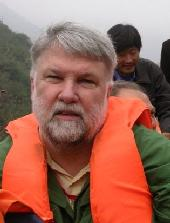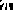# Ralph Howard

## University of South Carolina

```
```

Current classes: -
Mathematics 532/736I, Modern Geometry.
Office Hours: - 2:30pm--3:30pm, and by appointment.

## Previous classes and other class related material.

Research Interests: Differential and integral geometry with excursions into analysis, convexity, and mathematical relativity.

## Seminar and class notes:

• Analysis on Homogeneous Spaces.
Notes from a class titled Harmonic Analysis on Homogeneous Spaces given at the Royal Institute of Technology, Stockholm in the spring of 1994. They are elementary, incomplete, and disorganized, but redeem themselves by having lots of miss-prints and an index.
• A Question whose Answer is 42.
A straight tunnel is cut through the Earth, but not necessary though its center. Arthur Dent starts at rest at one end of the tunnel and slides, without friction or air resistance and only under the force of gravity, until he reaches the other end of the tunnel. Question: How long does the trip take Arthur?
• Customizing Planets to Control Weight.
Given a planet of uniform density and a point on its surface, how can we reshape the planet to maximize the gravitational force at the given point.
• The Milnor-Rogers proof of the Brouwer Fixed Point Theorem.
An exposition of the analytic proof of Milnor, as simplified by Rogers, of the Brouwer Fixed Point Theorem. All that is required is the inverse function theorem and the change of variable formula for multiple integrals.
• A counterexample to one of my favorite conjectures.
For a smooth compact manifold M let S(M) be the space of infinitely differentiable real valued functions on M. Given two manifolds M and N with dim(M)> dim(N) it is shown that there are injective linear linear maps of finite order from S(M) to S(N). I had conjecture that all such maps have infinite dimensional kernel, which, if it had been true, would have explained many non-injectiveity results in integral geometry.
• Generic Cut Loci are Dense.
If the set C of compact subsets of Rn is given made into a metric space using the Hausdorff distance, then set of K in C with dense cut locus dense in Rn is a dense G-delta set in C.
• Fubini's Theorem on the Termwise Differentiable of Series with Monotone Terms.
If f(x)=f1(x)+f2(x)+f3(x)+... is a pointwise convergent series of function each of which is monotone increasing, then we give a proof of Fubini's result that the derivative is given by f '(x)=f1'(x)+f2'(x)+f3'(x)+... almost everywhere. This is used to an easy example of function that is continuous, strictly increasing, but has derivative zero almost everywhere.
• Mohammad Ghomi's Solution to the Illumination Problem.
An exposition of a recent result of Mohammad Ghomi who has shown that if M is smooth compact oriented surface in R3 so that all the shadow sets of M are simply connected, then M is the boundary of a convex set. Also given is his example showing that "simply connected" can not be weakened to "connected".
• Characterization of Tantrix Curves.
Let c(t) be a regular curve in the Euclidean space R3. Then the tantrix is the curve on the sphere S2 given by t(t)=c'(t)/|c'(t)|. A proof of a folk theorem characterizing the tantrix curves of closed curves is given and extended to higher dimensions and the case of curves symmetric with respect to a group action.
• The Geometry of Shadow Boundaries on Surfaces in Space.
If a light is shined on a surface, the boundary between the light and the shadow is the shadow curve. These are notes, at an elementary level, on the geometry of shadow curves.
• Alexandrov's Theorem on the Second Derivatives of Convex Functions.
Notes giving a detailed proof of Alexandrov's Theorem that a convex suction has second derivatives almost everywhere. This done by use of Rademacher's theorem (a Lipschitz function between Euclidean spaces has first derivatives almost everywhere) whose proof is also included.
• John's Theorem on Ellipsoids in Convex Bodies.
A proof is given of the result of Fritz John that if K is a convex body in Rn and E is the ellipsoid of maximum volume in K then K is contained in c+n(K-c). When K is symmetric about the origin this can be improved so that K is contained in n1/2E.
• Notes on Extremal Approximately Convex Functions and the Size of Convex Hulls.
An expanded version of a preprint above (written with Steve Dilworth and Jim Roberts). The differences are extra figures, some exposition of results in the literature, and an alternate proof of one theorem.
• Upper Bounds for the Concentration Function in Combinatorial Number Theory.
Some notes based on lectures of Kostya Oskolkov giving a simplified proof of a theorem of Halász bounding the number of points a "random" sum of a set of n vectors, not clustered about any hyperplane, that can lie in a sufficiently small set.
• The Gronwall Inequality for Solutions to Ordinary Differential Equations.
A version of the Gronwall inequality that estimates the difference between solutions to two differential equations in terms of the difference between their initial conditions and how how much the two equations differ.
• The Loewner-Pu Inequality, Isosystolic Constants, and Quasi-Conformal Geometry.
The Loewner-Pu inequality is generalized to Riemannian metrics g on an n dimensional torus Tn that are C-quasi-conformal to a flat metric where the resulting inequality relates C and some isosytolic constants. For n > 2 this can be combined with recent results of Babenko and Katz to give examples of smooth metrics that have arbitrarily large "quasi-conformal distance" from the set of flat metrics on Tn.
• The Sharp Sobolev Inequality on Surfaces.
This is a preliminary and expanded version of one of the preprints above. It also contains an extra section giving a strengthened version of McKean's lower bound on the first eigenvalue of the Laplacian on a negatively curved surface.
• The Inverse Function Theorem for Lipschitz Maps.
This is an edited version of a proof, in the from of exercises with detailed hints, of the classical inverse function and the inverse function theorem for Lipschitz maps between Banach spaces that was given to a graduate class in differential equations as homework.
• Closed Riemannian Manifolds without Conjugate Points.
A self contained proof of the theorem of E. Hopf that a Riemannian metric on the two dimensional torus without conjugate points is flat. An extension to higher dimensions, due to L. Green, is also given. The proof follows that of Green.
• Gunther's Proof of the Nash Isometric imbedding Theorem.
A very elementary account of the local version of the Nash isometric embedding theorem using the method of Gunther.
• Kuiper's Theorem on Conformally Flat Compact Manifolds.
A proof of the result of Kuiper that a compact simply connected locally conformal flat Riemannian manifold is globally conformally equivalent to the standard sphere. The proof here, while it follows the basic outline of Kuiper's proof, requires less smoothness of the metric.
• Estimates on the Graphing Radius of Submanifolds and the Inradius of Domains.
If all principle curvatures of a complete hypersurface M of Euclidean space Rn have absolute value at most one then at any point of M the graphing radius is at least one. This is used to prove results on the inradius of domains in terms of the topology and the size of the principle curvatures.
• A Rigidity Theorem for Convex Surfaces.
If M is a two dimensional sphere with a Riemannian metric that has curvature in the interval [0,1] then every simple closed geodesic of M has length at least 2with equality iff M is either a standard sphere or a capped cylinder.

## Dissertations of Students:

• Somasundaram Velummylum:
The Calculus of Variations and Zoll Surfaces.
Master's Degree 1990.
• Shiying Zhao:
On the Boundary Behavior of Subharmonic Functions in Nontangential Acessible Domains.
Ph.D 1991. (Supervised jointly with Manfred Stoll.)
• Louis Kenneth Reinitz:
The Periodic Symmetry Groups of the Plane.
Master's Degree 1991.
• Gary Allen Cohen:
Intersection Theory.
Master's Degree 1991.
• Aseem Raval:
Mathematics Related to Spinning Tops.
Master's Degree 1999.
• Michael S. Venn:
Analysis on Finite Gel'fand Spaces.
Master's Degree 2001.
• Anamaria Rusu:
Determining Starlike Bodies by Their Curvature Intgrals.
Ph.D 2008.

## Contact information:

E-mail:
howard@math.sc.edu
Office:
LeConte 304
Mail:
Ralph Howard
Department of Mathematics
University of South Carolina
Columbia, SC 29208
USA
Telephone:
(803) 777-7471
FAX:
(803) 777-3783